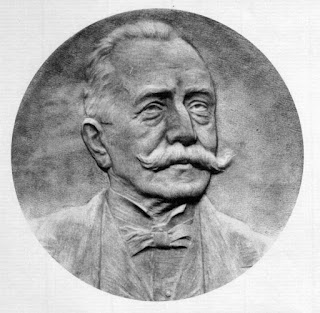## Posts

Showing posts from October, 2017

### Arzela-Ascoli 定理$\textbf{Bolzano-Weierstrass Theorem}$
$\mathbb{R}^n$ 裡的任何 $bounded\ sequence$ 必存在收斂 $subsequence$

$\underline{proof\ sketch}$

$\textbf{Compact}$

$\textbf{Uniform Continuous}$
$f$ 將 $X$ 映射到 $\mathbb{R}$ 是均勻連續（$Uniform\ Continuous$）$\iff$ 對任意 $\epsilon >0$，存在 $\delta >0$ 使得對任何 \$…